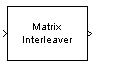# Matrix Interleaver

Permute input symbols by filling matrix by rows and emptying it by columns

## Library

Block sublibrary of Interleaving

•## Description

The Matrix Interleaver block performs block interleaving by filling a matrix with the input symbols row by row and then sending the matrix contents to the output port column by column.

The Number of rows and Number of columns parameters are the dimensions of the matrix that the block uses internally for its computations.

This block accepts a column vector input signal. The number of elements of the input vector must be the product of Number of rows and Number of columns.

The block accepts the following data types: `int8`, `uint8`, `int16`, `uint16`, `int32`, `uint32`, `boolean`, `single`, `double`, and fixed-point. The output signal inherits its data type from the input signal.

## Parameters

Number of rows

The number of rows in the matrix that the block uses for its computations.

Number of columns

The number of columns in the matrix that the block uses for its computations.

## Examples

If the Number of rows and Number of columns parameters are 2 and 3, respectively, then the interleaver uses a 2-by-3 matrix for its internal computations. Given an input signal of `[1; 2; 3; 4; 5; 6]`, the block produces an output of `[1; 4; 2; 5; 3; 6]`.

## Pair Block

Matrix Deinterleaver

## Version History

Introduced before R2006a